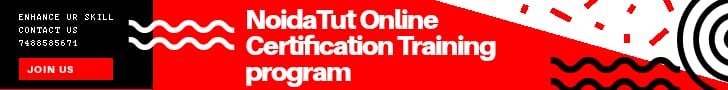FREE E LEARNING PLATFORM

# Number of Vowels and Consonants in a StringThis program takes the string entered by user and finds the number of vowels and consonants in the input string.

### Example: Program to find and display the number of vowels and Consonants in given String

```#include <iostream>
using namespace std;
int main(){
char str;
int vowelCounter = 0, consonantCounter = 0;
cout << "Enter any string: ";
cin.getline(str, 150);
//'\0 represent end of string
for(int i = 0; str[i]!='\0'; i++) {
if(str[i]=='a' || str[i]=='e' || str[i]=='i' || str[i]=='o' || str[i]=='u' || str[i]=='A' || str[i]=='E' || str[i]=='I' || str[i]=='O' || str[i]=='U')
{
vowelCounter++;
}
else if((str[i]>='a'&& str[i]<='z') || (str[i]>='A'&& str[i]<='Z'))
{
consonantCounter++;
}     }
cout << "Vowels in String: " << vowelCounter << endl;
cout << "Consonants in String: " << consonantCounter << endl;
return 0;  }```

### Output

```Enter any string: Hello Guys, Welcome to Beginnersbook.com
Vowels in String: 13
Consonants in String: 21```

The same program can be written by using Strings in C++. To use string in place of char array, replace the following part of the code in above program:

```char str;
int vowelCounter = 0, consonantCounter = 0;
cout <<
"Enter any string: ";
cin.getline(str, 150);
```
With This
```string str;
int vowelCounter = 0, consonantCounter = 0;
cout << "Enter any string: ";
getline(cin, str);```